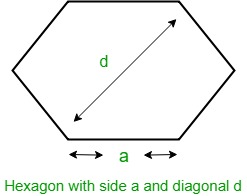Open In App

# Area of hexagon with given diagonal length

You are given the length of the diagonal of a hexagon, d. Your task is to find the area of that hexagon.
Examples:

Input :   5
Output :   Area of Hexagon: 16.238

Input : 10
Output : Area of Hexagon: 64.9519

Hexagon
Hexagon is a regular polygon having six equal sides and all equal angles. The interior angles of Hexagon are of 120 degrees each and the sum of all angles of a Hexagon is 720 degrees.Let d be the diagonal of Hexagon, then the formula to find the area of Hexagon given by
Area =How does above formula work?
We know that area of hexagon with side length a = (3 √3(a)2 ) / 2. Since all sides are of same size and angle is 120 degree, d = 2a or a = d/2. After putting this value, we get area as (3 √3(d)2 ) / 8.
Below is the implementation .

## C++

 // C++ program to find the area of Hexagon with given diagonal#include using namespace std; // Function to calculate areafloat hexagonArea(float d){    // Formula to find area    return (3 * sqrt(3) * pow(d, 2)) / 8;} // Mainint main(){    float d = 10;    cout << "Area of hexagon: " << hexagonArea(d);    return 0;}

## Java

 // Java program to find the area of// Hexagon with given diagonalimport java.lang.Math; public class GfG {     // Function to calculate area    public static float hexagonArea(float d)    {        // Formula to find area        return (float)((3 * Math.sqrt(3) * d * d) / 8);    }     public static void main(String []args) {        float d = 10;        System.out.println("Area of hexagon: " + hexagonArea(d));    }} // This code is contributed by Rituraj Jain

## Python3

 # Python3 program to find the area# of Hexagon with given diagonalfrom math import sqrt # Function to calculate areadef hexagonArea(d) :     # Formula to find area    return (3 * sqrt(3) * pow(d, 2)) / 8 # Driver codeif __name__ == "__main__" :     d = 10    print("Area of hexagon:",           round(hexagonArea(d), 3)) # This code is contributed by Ryuga

## C#

 // C# program to find the area of// Hexagon with given diagonal using System; public class GFG{         // Function to calculate area    public static float hexagonArea(float d)    {        // Formula to find area        return (float)((3 * Math.Sqrt(3) * d * d) / 8);    }  //Code driven    static public void Main (){            float d = 10;        Console.WriteLine("Area of hexagon: " + hexagonArea(d));    }//This code is contributed by Tushil.   }

## PHP

 

## Javascript

 

Output:

Area of Hexagon:  64.952

Time Complexity: O(1)

Auxiliary Space: O(1), since no extra space has been taken.